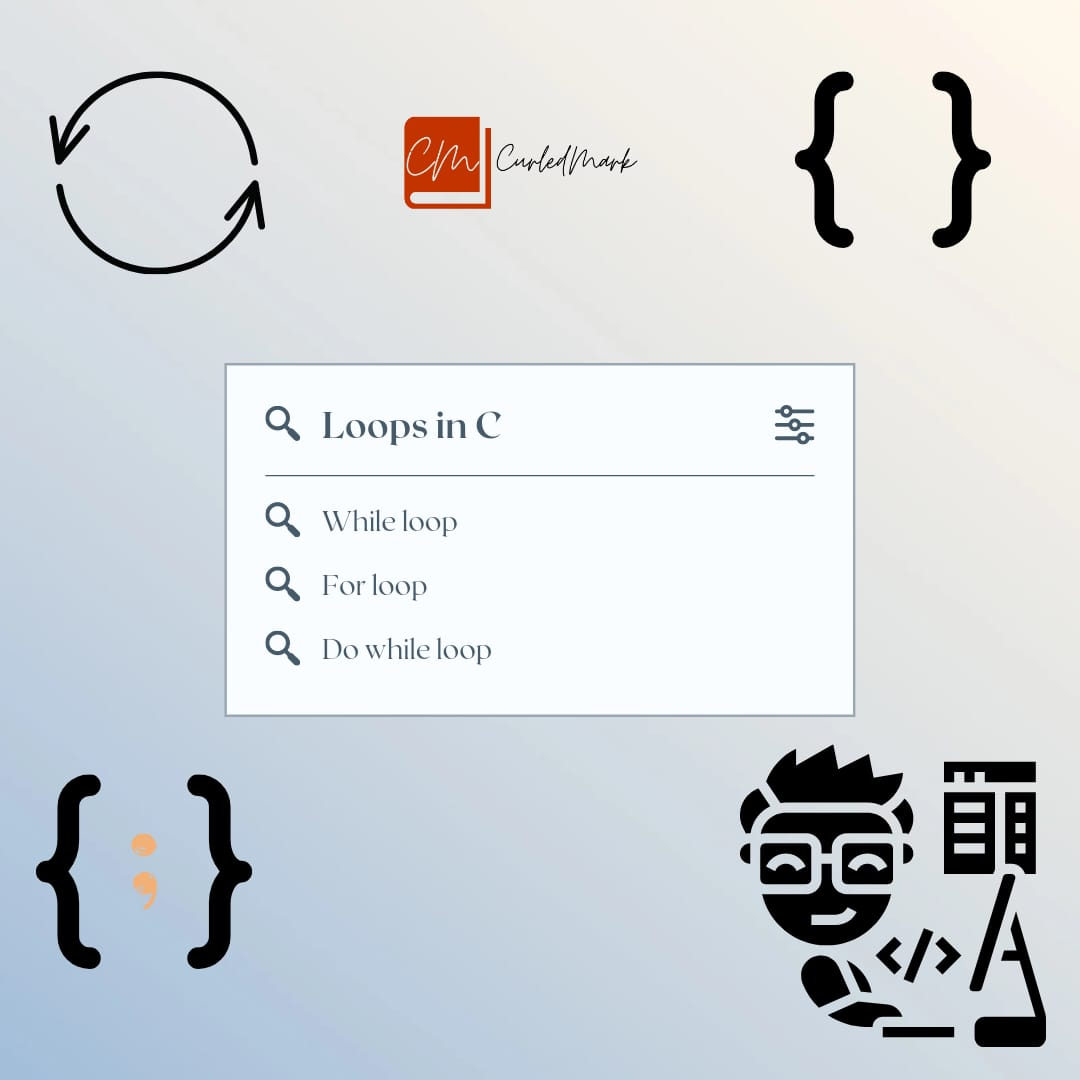September 26, 2022Software developer programming code on computer. Abstract computer script source code.

# How to write a prime number Program in C

A prime number is a positive integer that is divisible only by 1 and itself. For example: 2, 3, 5, 7, 11, 13, 17. The Prime number program is a program where we find out whether the number is prime(is greater than 1 and divided by 1 or itself) or not.
1. Introduction
2. The logic of the Prime number in C
3. Program of Prime Number in C
4. Output
5. Explanation

## Introduction of Prime number Program

Prime number in C: A prime number is a number that is greater than 1 and divided by 1 or itself. In other words, prime numbers can’t be divided by other numbers than itself or 1. For example 2, 3, 5, 7, 11, 13, 17, 19, 23…. are the prime numbers.

## The logic of Prime number Program

while( y<=ep)
{
if(n%y==0)
{
z=1;
break;
}
y++;
}
if(z==0)
{
printf(“%d is a prime number”,n);
}
else
{
printf(“%d is not a prime number”,n);
}

## Program of Prime Number in C in Notepad

#include<stdio.h>
int main()
{
int n, y, ep, z;
printf(“Enter a number: “);
scanf(“%d”,&n);
if(n<=1)
{
printf(“%d is neither prime nor composite”);
return 0;
}
ep=n/2;
z=0;
y=2;
while( y<=ep)
{
if(n%y==0)
{
z=1;
break;
}
y++;
}
if(z==0)
{
printf(“%d is a prime number”,n);
}
else
{
printf(“%d is not a prime number”,n);
}
return 0;
}

## Output in cmd

D:\C Examples>prime.exe
Enter a number: 11
11 is a prime number
D:\C Examples>prime.exe
Enter a number: 9
9 is not a prime number
D:\C Examples>prime. exe
Enter a number: -1
6422288 is neither prime nor composite
D:\C Examples>prime. exe
Enter a number: 13
13 is a prime number
D:\C Examples>

## Explanation

Firstly, in the program, we have accepted the number from keyboard. As we know negative numbers and zero is not a prime number so we have put that condition that if the number is less than 1 then it is neither composite or prime. In the while loop, we have checked from 2 to ep where ep=ep/2. In the while condition, there is an if condition which checks when n is divided by y and we get 0 then z=1, which means the number is not prime. Here is the flag variable which indicates 1 or 0. If it is 1 then it is not prime and if it is 0 then it is prime.

So, the last condition is checked via if that it is prime or not.

### Click on the below link to check how we can run program in command prompt

How to Run C Program in Command Prompt

## Reference

https://curledmark.in/the-largest-number-program-in-an-array-in-c-programming/

https://curledmark.in/armstrong-number-and-arrays-in-c-programming/

https://curledmark.in/prime-reversepower-programs-in-c-programming/

The Book Of Five Rings Summary

The Blue Umbrella

How to Talk to Anyone by Leil Lowndes

Jonathan Livingston Seagull Book Summary

Your Brain on PORN Book Summary by Gary Wilson

THE IMMORTALS OF MELUHA By Amish Tripathi full summary

Eleven Minutes by Paulo Coelho Summary

#### You may have missed#### What is do-while loop in C Programming ? Loops